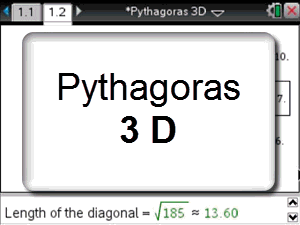# Activities

•• ##### Subject Area

• Standard: National Curriculum 7-10: Measurement and Geometry: Shape
• Standard: National Curriculum 7-10: Measurement and Geometry: Geometric reasoning

• ##### AuthorMiddle

60 Minutes

• ##### Device
• TI-Nspire™ CAS
• ##### Software

TI-Nspire™ CAS

3.2

## Pythagoras 3D#### Activity Overview

Students use an interactive diagram of a three dimensional box to explore the relationship between the side lengths of the box and the length of the diagonal connecting opposing vertices. Students verify the length of the diagonal by using Pythagoras’s theorem in three dimensions.

#### Objectives

ACMMG276 - Pythagoras' theorem and trigonometry to solving three dimensional problems in right-angled triangles

• Diagonal
• Hypotenuse
• Pythagoras